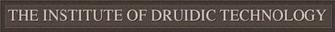# Unary Numbers

The basics of the unary number system, the simplest of arithmetic bases, is quite elementary. Whereas the more complicated binary number system uses two digits; the simpler unary number system uses one.

Therefore we have the following decimal -> unary identities:

```    1  =  |
2  =  ||
3  =  |||
```

These first three digits appear to have been copied by the Romans (perhaps from early contact with the Gauls?), however, here the Celts diverge with a more elegant accounting:

```    4  =  ||||
5  =  |||||
6  =  ||||||
7  =  |||||||
8  =  ||||||||
9  =  |||||||||
10 =  ||||||||||
```

And now, we hold our breath. In all previous ancient cultures -- Sumer, Babylonia, Egypt -- there is a direct one-to-one correspondance between the ten fingers and the ten digits. All these cultures remained intractably grounded in the here and now. But here, finally, some anonymous Celt made the transcendental shift from the concrete to the abstract:

```    11 =  |||||||||||
12 =  ||||||||||||
13 =  |||||||||||||
(etc.)
```

You are probably wondering, what is the origin of the symbol "|"? You might think it is the finger, but no - it is the trunk of the birch tree -- prized as sacred by the Celts because of its fundamental role in the original megalithic rod memories used at Stonehenge.

Contact Dr. Tunalu at tunalu@jbum.com